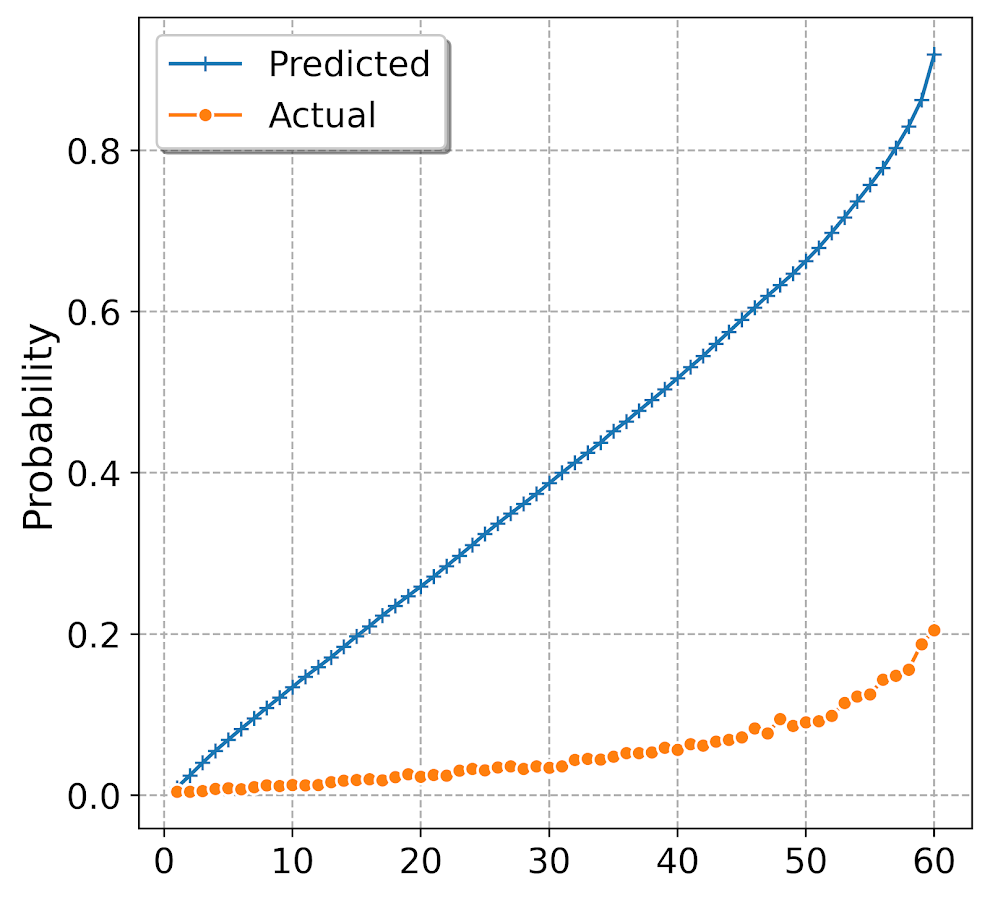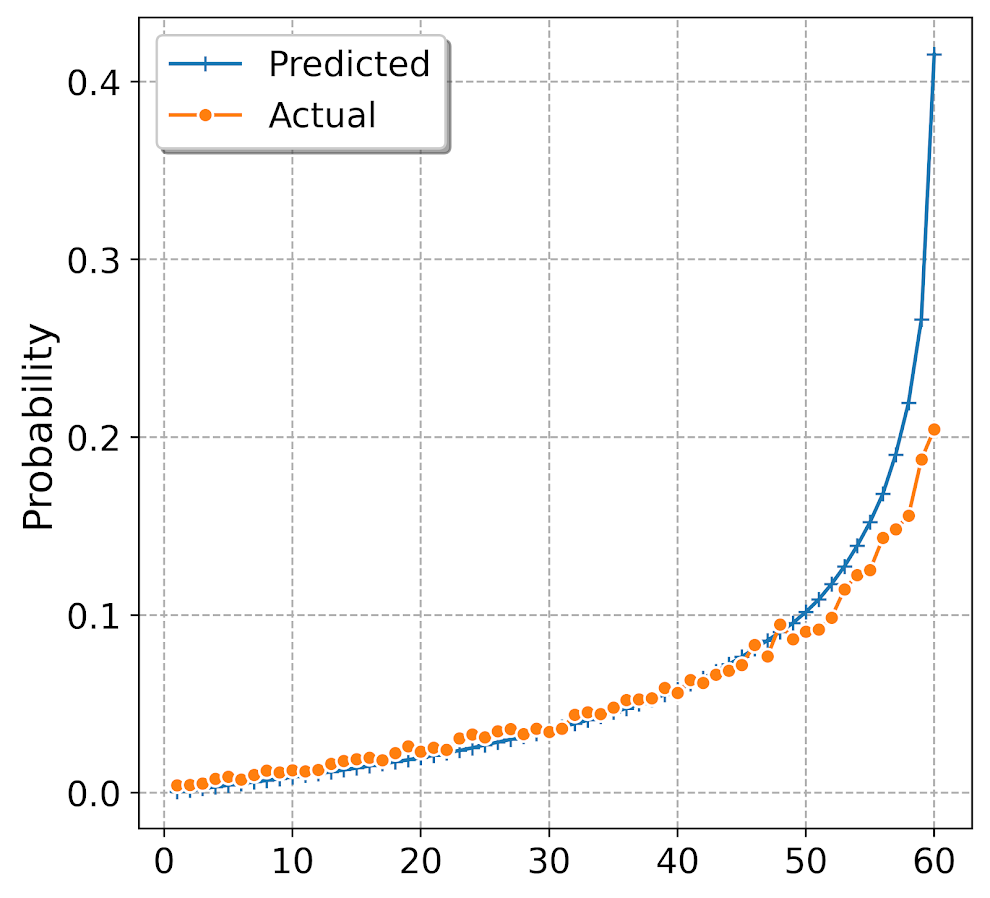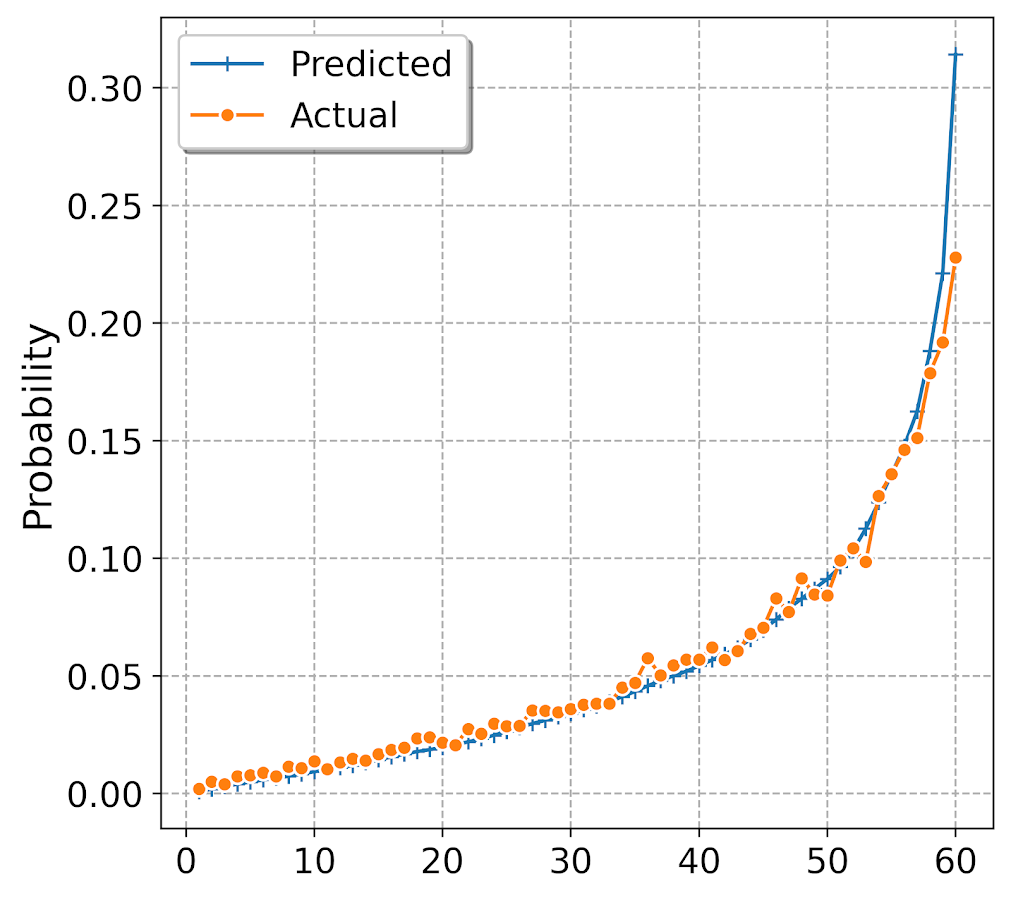# Adjusting predicted probabilities for sampling

Ryx had a blog post the other day about how many confuse how to turn predicted probabilities into a final classification 0/1 decision, Why Do So Many Practicing Data Scientists Not Understand Logistic Regression?. (Highly suggest Ryx’s blog and twitter feed in general, opinions expressed frequently mirror my own very closely.)

So I won’t go into why SMOTE (synthetic upsampling of the rare class) in general doesn’t make sense for most predictive applications here. (It may in some complicated machine learning scenarios I don’t fully understand.) But, there are a few scenarios where downsampling makes total sense. One is in case control studies, where it is costly to sample the control cases. (Motivated in part to write this as I reviewed a paper the other day that estimated marginal effects on the probability scale using case-control data, so they need to adjust them using the same technique I show here.) The other scenario, which I expect is more common for the working data scientist, is you just have a crazy large dataset, so you need to downsample just to fit the model of interest.

Say you are looking at fraud in bank transactions, and you have 500 million transactions and only 500,000 identified fraud cases. It makes total sense to take a sample of 500,000 control cases and then fit your models on the cases vs controls using whatever complicated model you want just so you can get an answer in a decent time on your local machine.

The predicted probabilities from that adjusted sample though will be wrong. But fortunately it is quite easy to adjust them back to the scale you want (and this will work just as well for SMOTE upsampling as well). I illustrate using an example in python and XGBoost. Most examples online show this for GLMs, but it works the same way for any model that returns a predicted probability.

So first lets load our libraries and create some simulated data. Here the positive class only occurs around 5% of the time.

``````#############################################
import numpy as np
import pandas as pd
import matplotlib
import matplotlib.pyplot as plt
from xgboost import XGBClassifier

#Creating simulated data

np.random.seed(10)
total_cases = 1000000
x1 = np.random.uniform(size=total_cases)
x2 = np.random.binomial(1,0.5,size=total_cases)
x3 = np.random.poisson(5,size=total_cases)
y_logit = -1 + 0.3*x1 + 0.1*x2 + -0.5*x3 + 0.05*x1*x2 + -0.03*x2*x3
y_prob = 1/(1 + np.exp(-y_logit))
y_bin = np.random.binomial(1,y_prob)
my_vars = ['x1','x2','x3','y_prob','y_bin']
sim_data = pd.DataFrame(zip(x1,x2,x3,y_prob,y_bin),
columns=my_vars)
x_vars = my_vars[:3]
y_var = my_vars[-1]

#Checking the distribution, make intercept larger if
#Not enough 0's to your liking
print( sim_data[y_var].mean() )
sim_data['y_prob'].hist(bins=100)
#############################################``````

The model is not that complicated (just some interactions), so hopefully XGBoost has no problem fitting it. But say we are in the ‘need to downsample because my data is too big scenario’. So here I create a training dataset that has a 50/50 split for the positive negative cases, and keep the test data the same.

``````#############################################
#Creating test/train samples

sim_data['index'] = range(sim_data.shape)
train = sim_data[sim_data['index'] <= 700000]
test = sim_data[sim_data['index'] > 700000]

#downsampling the training dataset
#so classes are equal
down_n = train['y_bin'].sum()
down_prop = train['y_bin'].mean()
down_neg = train[train['y_bin'] == 0].sample(n=down_n)
down_pos = train[train['y_bin'] == 1]
train_down = pd.concat([down_neg,down_pos],axis=0)

wrong_pr =  train_down[y_var].mean()
print( wrong_pr )
#############################################``````

So if you are following along in python, `wrong_pr` here is exactly 0.5 by construction. So next we fit our XGBoost model, generate the predicted probabilities on the test dataset, and then draw a lift-calibration chart. (If you are not familiar with what XGBoost is, I suggest this statquest series of videos. You can just pretend it is a black box here though that you get out predicted probabilities.)

``````#############################################
#Upping the number of trees for a higher resolution of
#predicted probabilities
model = XGBClassifier(n_estimators=1000)
model.fit(train_down[x_vars], train_down[y_var])

#making predictions for the test set
#probabilities
y_pred = model.predict_proba(test[x_vars])
test['y_pred_down'] = y_pred[:,1]

#Now looking at the calibration
def cal_data(prob, true, data, bins, plot=False, figsize=(6,4), save_plot=False):
cal_dat = data[[prob,true]].copy()
cal_dat['Count'] = 1
cal_dat['Bin'] = pd.qcut(cal_dat[prob], bins, range(bins) ).astype(int) + 1
agg_bins = cal_dat.groupby('Bin', as_index=False)['Count',prob,true].sum()
agg_bins['Predicted'] = agg_bins[prob]/agg_bins['Count']
agg_bins['Actual'] = agg_bins[true]/agg_bins['Count']
if plot:
fig, ax = plt.subplots(figsize=figsize)
ax.plot(agg_bins['Bin'], agg_bins['Predicted'], marker='+', label='Predicted')
ax.plot(agg_bins['Bin'], agg_bins['Actual'], marker='o', markeredgecolor='w', label='Actual')
ax.set_ylabel('Probability')
ax.legend(loc='upper left')
if save_plot:
plt.savefig(save_plot, dpi=500, bbox_inches='tight')
plt.show()
return agg_bins

cal_down = cal_data(prob='y_pred_down',true=y_var,data=test,bins=60,
plot=True, figsize=(6,6))
#############################################``````Oh my, you can see that our calibration is very bad. I am predicting something to happen 80% of the time in the top bin, but in reality it only happens 20% of the time. But we can fix it using an adjustment formula (originally saw the idea from Norm Matloff and rewrote an R function to python).

``````#############################################
#Rebalancing function

#rewrite from
#https://github.com/matloff/regtools/blob/master/inst/UnbalancedClasses.md
#and https://www.listendata.com/2015/04/oversampling-for-rare-event.htm
a = condprobs/(wrongprob/trueprob)
comp_cond = 1 - condprobs
comp_wrong = 1 - wrongprob
comp_true = 1 - trueprob
b = comp_cond/(comp_wrong/comp_true)
return a/(a+b)

plot=True, figsize=(6,6))
#############################################``````Alright, now we are much closer. It still is overpredicting at the highest classes (predicts 40% of the time when it should predict only 20%), but it is well calibrated for low predictions.

For kicks, I estimated the XGBoost model using the original sample data that is unbalanced and calculated the calibration plot.

``````#############################################
#What does it look like if I train with original?

model2 = XGBClassifier(n_estimators=1000)
model2.fit(train[x_vars], train[y_var]) #takes a minute!

#making predictions for the test set
#probabilities
y_pred = model2.predict_proba(test[x_vars])
test['y_pred_orig'] = y_pred[:,1]

plot=True, figsize=(6,6))
#############################################``````So this is slightly better than the adjusted downsampled XGBoost, but it still shows it is overpredicting for the tail of the dataset. But the overprediction here is like 31% vs 23%, where prior we were talking about 40% vs 20%.

Why these calibration metrics matter is that to generate estimates of how much money your model is making in practice will almost always rely on correctly estimating predicted probabilities, which translate into true positives and false negatives. If the model is not well calibrated, your estimates of these will be gravely off.

It doesn’t always matter, these transformations don’t change the rank order of the predictions. So say you are always just grabbing the top 100 predictions, this adjustment does not change what predictions are in the top 100. It will change how many of those cases though you expect to translate into true positives though.